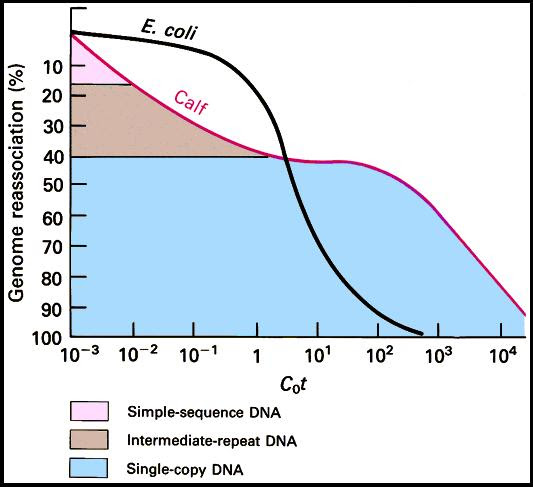# Cot value and Cot Curve analysis

It is a technique for measuring the complexity (size) of DNA or genome. The technique was developed by Roy Britten and Eric Davidson in 1960. The technique is based on the principle of DNA renaturation kinetics.

Principle:
• The rate of renaturation is directly proportional to the number of times the sequences are present in the genome.
• Given enough time all DNA that is denatured will reassociate or reanneal in a given DNA sample.
• The more the repetitive sequence the less will be the time taken for renaturation.
Procedure : The process involves denaturation of DNA by heating and allowed to reanneal by cooling. The renaturation of DNA is assessed stereoscopically. Large DNA molecules take longer time to reanneal.

What is cot value?
The renaturation depends on the following factors DNA concentration, reassociation temperature, cation concentration and viscosity.

Cot=DNA Concentration (moles/L) X renaturation time in seconds X buffer factor (that accounts for the effects of cations on the speed of renaturation).
Cot:Co=Concentration of DNA and t= time taken for renaturation
Low cot value indicates more number of repetitive sequences
High cot value indicates more number of unique sequences or less number of repetitive sequences.For example:
Bacteria- 99.7% Single Copy
Mouse - 60% Single Copy +25% Middle Repetitive+ 10% Highly Repetitive

How to calculate cot value?
Cot=DNA Concentration (moles/L) X renaturation time in seconds X buffer factor (that accounts for the effects of cations on the speed of renaturation).
Nucleotide concentration = 0.050 M
Renaturation time = 344 sec
Buffer factor, 0.5 M SPB = 5.820
Cot value = 0.050X 344 X 5.820=100.000
Applications of Cot curve analysis:• Understanding genome size and complexity
• Understanding complexity of sequences
• Understanding relative proportion of single copy and repetitive sequences